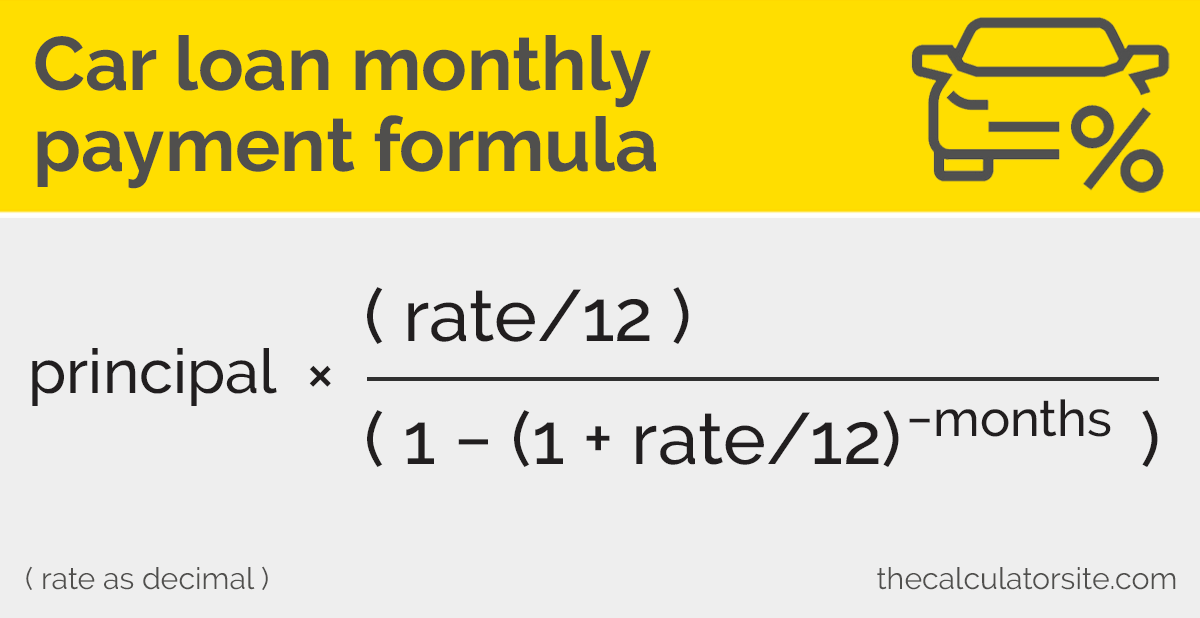# Car Loan Payoff Calculator


$Calculate interest on a car/auto loan and work out monthly repayment figures, interest splits and payoff schedules. Disclaimer: Whilst every effort has been made in building our calculator tools, we are not to be held liable for any damages or monetary losses arising out of or in connection with their use. Full disclaimer. ## How to calculate interest on a car loan 1. Calculate the monthly payment using the monthly payment formula. 2. Multiply the monthly payment by the number of months the loan is for, to get the total repayment amount. 3. Deduct the principal amount from your total repayment amount to get the total interest.### Step by step instructions Step 1: let's calculate the monthly payment using the monthly payment formula. When using this formula, remember that 'rate' needs to be represented as a decimal (rather than as a percentage). $$principal \times \dfrac{(rate/12)}{(1 - (1 + rate/12) ^{-months} )}$$ Here's an example of a$15000 car loan at 6% for 60 months.

$$15000 \times \dfrac{(0.06/12)}{(1 - (1 + 0.06/12) ^{-60} )}$$
$$15000 \times \dfrac{0.005}{1 - (1.005 ^{-60} )}$$
$$\dfrac{75}{0.2586278} = 289.992$$

Step 2: We now have our monthly payment figure ($289.99). To calculate the total repayment amount, multiply this figure by the length of the loan (60 months): $$289.992 \times 60 = 17399.52$$ Step 3: To calculate the total interest on the car loan, deduct your principal figure from the total repayment figure: $$17399.52 - 15000 = 2399.52$$ For this example, the total interest on the car loan is$2399.52. You can check this figure with the car loan payoff calculator at the top of this page.

## How does the car loan payoff calculator work?

Our calculator helps you work out the costs associated with purchasing a car on credit. Once you have entered the amount, the interest rate and the period of the loan, the calculator will return the total repayment amount, the total interest and the monthly payment figure, as well as full amortization.

## How much interest will I pay on my car loan?

Our car finance calculator works out the interest that you might pay as part of your car finance plan. It does this by taking your interest rate and compounding it over the course of the loan period. It is this compounding of interest rate that forms the basis of the effective annual rate we feature in our calculator. You may be interested to note that we have a calculator for compounding interest on savings.## Why take out a car loan?

When it comes to financing a new car, there are a number of options available to you: outright purchase, personal loan, leasing, hire purchase or dealer financing. It's advisable to read up on the pros and cons of each of these before deciding upon the best one for you. Should you be considering taking out a different type of loan, give our standard loan calculator a try. You can even include extra payments in your calculation.

## What is a balloon payment?

A balloon payment is a large, lump-sum payment made at the end of a long-term loan. It is commonly used in car finance loans as a way of reducing monthly repayment figures. Be aware that once you reach the end of your loan period, the balloon amount becomes payable. You can learn more about this subject in our featured article about balloon payments.

From abacus to iPhones, learn how calculators developed over time.